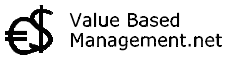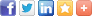## Weighted Average Cost of CapitalCategories: Articles  |  Books  |  Dictionary  |  Faq  |  Home

# WACC

Investment analysis:

## Summary of WACC - Weighted Average Cost of Capital. Abstract

Corporations create value for shareholders by earning a return on the invested capital that is above the cost of that capital.  WACC (Weighted Average Cost of Capital) is an expression of this cost and is used to see if certain intended investments or strategies or projects or purchases are worthwhile to undertake.

WACC is expressed as a percentage, like interest. So for example if a company works with a WACC of 12%, than this means that only (and all) investments should be made that give a return higher than the WACC of 12%.

The cost of capital for any investment, whether for an entire company or for a project, is the rate of return capital providers would expect to receive if they would invest their capital elsewhere. In other words, the cost of capital is an opportunity cost.

How can the Weighted Average Cost of Capital (WACC) be calculated?

The easy part of WACC is the debt part of it. In most cases it is clear how much a company has to pay their bankers or bondholders for debt finance. More elusive however, is the cost of equity finance. Normally, the cost of equity finance is higher than the cost debt finance, because the cost of equity involves a risk premium. Calculating this risk premium is one thing that makes calculating WACC complicated.

Another important complication is which mix of debt and equity should be used to maximize shareholder value (This is what "Weighted" means in WACC).

Finally, also the corporate tax rate is important, because normally interest payments are tax-deductible.

### Formula WACC Calculation

debt / TF (cost of debt)(1-Tax)

+    equity/ TF (cost of equity)

---------------------------------------------------------

WACC

In this formula,

* TF means Total Financing. Total Financing consists of the sum of the Market values of debt and equity finance. An issue with TF is whether, and under what circumstances, it should include current liabilities, such as trade credit. In valuing a company this is important, because: a) trade credit is used aggressively by many companies, which in turn affects their business credit, b) there is an interest (or financing) charge for such use, and c) trade credit can be quite a large sum on the balance sheet.

* Tax stands for the Corporate Tax Rate.

Example: suppose this company:

The Market value of debt = € 300 million

The Market value of equity = € 400 million

The Cost of debt = 8%

The Corporate Tax rate = 35%

The Cost of equity is 18%

The WACC of this company is:

300:700*8%*(1-35%)

+  400:700*18%

---------------------------------------------------------

12,5% (WACC - Weighted Average Cost of Capital)

Compare: IRR  |  Net Present ValueDCF

 ©2016 Value Based Management.net - Last updated: Jan 6th, 2016 - All names ™ by their owners